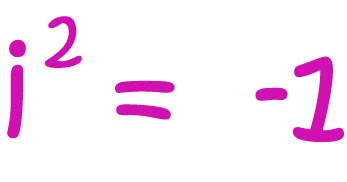# Definition of Imaginary NumberAn Imaginary Number is a number that squares to give a negative result.

The real numbers are not closed under taking square roots. You can't find real square roots of negative numbers.

Back in AD 50, a Greek mathematician, Heron of Alexandria, decided this was a bad thing, but didn't get very far with solving the problem. It wasn't until the 16th century that mathematicians gave this problem any serious attention. In the late 16th century, Rafael Bombelli came up with a notation for $\sqrt{-1}$, the imaginary number that we call $i$.

So, $i$ is the square root of $-1$.

Note that phsyicists and engineers sometimes call this number $j$.

The number system formed by adding $i$ to the real numbers is called the complex number system. It includes the square roots of all the negative numbers. The term, "imaginary number" was chosen because these numbers were originally viewed with great suspicion.

### Description

The aim of this dictionary is to provide definitions to common mathematical terms. Students learn a new math skill every week at school, sometimes just before they start a new skill, if they want to look at what a specific term means, this is where this dictionary will become handy and a go-to guide for a student.

### Audience

Year 1 to Year 12 students

### Learning Objectives

Learn common math terms starting with letter I

Author: Subject Coach
You must be logged in as Student to ask a Question.